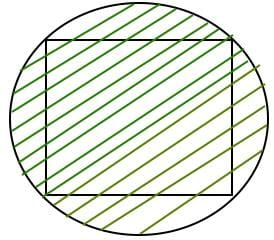GMAT  >  Test: Trigonometry- 2

# Test: Trigonometry- 2

Test Description

## 10 Questions MCQ Test Quantitative Reasoning for GMAT | Test: Trigonometry- 2

Test: Trigonometry- 2 for GMAT 2022 is part of Quantitative Reasoning for GMAT preparation. The Test: Trigonometry- 2 questions and answers have been prepared according to the GMAT exam syllabus.The Test: Trigonometry- 2 MCQs are made for GMAT 2022 Exam. Find important definitions, questions, notes, meanings, examples, exercises, MCQs and online tests for Test: Trigonometry- 2 below.
Solutions of Test: Trigonometry- 2 questions in English are available as part of our Quantitative Reasoning for GMAT for GMAT & Test: Trigonometry- 2 solutions in Hindi for Quantitative Reasoning for GMAT course. Download more important topics, notes, lectures and mock test series for GMAT Exam by signing up for free. Attempt Test: Trigonometry- 2 | 10 questions in 20 minutes | Mock test for GMAT preparation | Free important questions MCQ to study Quantitative Reasoning for GMAT for GMAT Exam | Download free PDF with solutions
 1 Crore+ students have signed up on EduRev. Have you?
Test: Trigonometry- 2 - Question 1

### What is the maximum value of 5 Sinθ + 12 cosθ?

Detailed Solution for Test: Trigonometry- 2 - Question 1

Maximum value = √(a2 + b2)
=13

Test: Trigonometry- 2 - Question 2

### What is minimum value of Sinθ + cosθ ?

Detailed Solution for Test: Trigonometry- 2 - Question 2

Minimum value = √(a2 – b2)
=-√2

Test: Trigonometry- 2 - Question 3

### If tan (x+y) tan (x-y) = 1, then find tan (2x/3)?

Detailed Solution for Test: Trigonometry- 2 - Question 3

tanA = cotB,
tanA*tan B = 1
So, A +B = 90o
(x+y)+(x-y) = 90o, 2x = 90o , x = 45o
Tan (2x/3) = tan 30o = 1/√3

Test: Trigonometry- 2 - Question 4

Find the Value of tan60o + tan120o?

Detailed Solution for Test: Trigonometry- 2 - Question 4

tan(60) + tan(180-120)

=tan(60)+(-tan(60)) {because in second quadrant tan is -ve}

=0

Test: Trigonometry- 2 - Question 5

The least value of 2sin2θ + 3cos2θ

Detailed Solution for Test: Trigonometry- 2 - Question 5

2sin2θ + 2cos2θ + cos2θ
=2(sin2θ + cos2θ) + cos2θ ; (by putting sin2θ + cos2=1)
= 2 + cos2θ ;(the minimum value of cos2θ=0)
= 2 + 0 = 2

Test: Trigonometry- 2 - Question 6

The angle of elevation of the sun, when the length of the shadow of a tree is √3 times the height of the tree, is:

Detailed Solution for Test: Trigonometry- 2 - Question 6

Let AB be the height of the tree and AC be the length of the shadow. We need to calculate the angle ACB where BC is the hypotenuse.
Given,
AC:AB = √3
cot θ = √3
θ = 30 degrees.

Test: Trigonometry- 2 - Question 7

From a point P on a level ground, the angle of elevation of the top of a tower is 30 degrees. If the tower is 100 m high, the distance of point P from the foot of the tower is:

Detailed Solution for Test: Trigonometry- 2 - Question 7

Let QR be the tower. Then,
QR = 100 m and angle QPR = 30 degrees.
We know, cot 30° = √3 = PQ/QR.
Therefore, PQ = 100*√3 = 173 m.

Test: Trigonometry- 2 - Question 8

A man standing at a point P is watching the top of a tower, which makes an angle of elevation of 30° with the man’s eye. The man walks some distance towards the tower to watch its top and the angle of elevation becomes 60°. What is the distance between the base of the tower and the point P?

Detailed Solution for Test: Trigonometry- 2 - Question 8

Let MN be the tower and man be standing at P (30° = angle MPN) and Q (60° = angle MQN). We are only given two angles and no sides of the triangles. Therefore, the data is inadequate.

Test: Trigonometry- 2 - Question 9

A man is watching from the top of a tower a boat speeding away from the tower. The boat makes an angle of depression of 45° with the man’s eye when at a distance of 60 meters from the tower. After 5 seconds, the angle of depression becomes 30°. What is the approximate speed of the boat, assuming that it is running in still water?

Detailed Solution for Test: Trigonometry- 2 - Question 9

Let the tower be PQ and the boat be at positions R and S when making angles of 45° and 30° respectively.
Given, PR = 60 m.
Now, PQ/PR = tan 45° = 1. So, PQ = PR = 60 m.
Again, PQ/PS = tan 30° = 1/√3. So, PS = 60 * √3 m = 103.92 m.
Distance covered in 5 seconds = 103.92 – 60 = 43.92 m.
Speed in kmph = (43.92/5) * (18/5) = 32 kmph (approximately)

Test: Trigonometry- 2 - Question 10

The area of a square is ‘d’. What is the area of the circle which has the diagonal of the square as its diameter?

Detailed Solution for Test: Trigonometry- 2 - Question 10Diagonal of Square = Diameter of Circle.

Let side of square be x.

From Pythogorous theorem.

Diagonal = √(2*x*x)

We know area of square = x * x = d ⇒ Diameter = Diagonal = √(2*d)

Area of Circle = π * √(d/2) * √(d/2)

= 1/2 * π * d

## Quantitative Reasoning for GMAT

75 videos|87 docs|156 tests
 Use Code STAYHOME200 and get INR 200 additional OFF Use Coupon Code
Information about Test: Trigonometry- 2 Page
In this test you can find the Exam questions for Test: Trigonometry- 2 solved & explained in the simplest way possible. Besides giving Questions and answers for Test: Trigonometry- 2, EduRev gives you an ample number of Online tests for practice

## Quantitative Reasoning for GMAT

75 videos|87 docs|156 tests